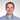# Stack Data Structure In JavaScript

July 11, 2018 - 5 min read

It’s not a secret that we can use JS arrays as stacks, but how about implementing it from scratch? It’s a good practice allowing us to better understand this data structure, use it in our daily job more thoughtfully and thus become better developers. Let’s dive into it, but first, let’s recall what the stack actually is.

# Stack

The stack is the data structure that manages a collection of elements with two main operations:

• push - which adds an element to the collection;
• pop - which removes the most recently added element from the collection.

We can think of the stack as a physical stack of objects, for example, a stack of books. Let’s remember this analogy, it will serve us later. The stack is also known as the LIFO (Last In First Out) structure - the last books we put on stack goes first out of it.

# Big-O Analysis

Here is the big-O analysis for the main operations:

• push(el) - O(1)
• pop() - O(1)

But what is the big-O analysis for the operation of popping the first element in the stack. Let’s recall our analogy with books and it becomes obvious that if we have a stack of N books then to be able to access the first book in the stack we have to pop the books from the top one by one N times. So, the big-O analysis for this operation is O(N).

# Stack in JavaScript

Just as a quick reminder and the reference, we can use our well-known JS arrays as stacks. Let’s use it to illustrate the situation with books stacking them first and unstacking then.

``````const stack = []

// Stacking books one on top of another
stack.push("The Philosopher's Stone")
stack.push("The Chamber of Secrets")
stack.push("The Prisoner of Azkaban")
stack.push("The Goblet of Fire")
stack.push("The Order of the Phoenix")
stack.push("The Half-Blood Prince")
stack.push("The Deathly Hallows")

// Unstacking books
stack.pop() // -> "The Deathly Hallows" | We put this book the last but take the first.
stack.pop() // -> "The Half-Blood Prince" | We put this book the previous to the last so we take it second``````

# Stack Implementation

Alright, let’s implement the stack by our own. We don’t want to emulate the implementation by creating a wrapper on top of the array. Instead, let’s do it from scratch.

## Preliminary Considerations

Let’s figure out first what we’d like to achieve. In other words, let’s imagine that we have already created the stack. How will we use it? Well, obviously, in the exact same manner as we did above:

``````const stack = new Stack()

// Stacking books one on top of another
stack.push("The Philosopher's Stone")
stack.push("The Chamber of Secrets")
stack.push("The Prisoner of Azkaban")
stack.push("The Goblet of Fire")
stack.push("The Order of the Phoenix")
stack.push("The Half-Blood Prince")
stack.push("The Deathly Hallows")

// Unstacking books
stack.pop() // -> "The Deathly Hallows" | We put this book the last but take the first.
stack.pop() // -> "The Half-Blood Prince" | We put this book the previous to the last so we take it second``````

Great! Now we have a clear vision what to implement. The next step is to figure out what will be the storage mechanism working under the hood of the stack. The good candidate is just a plain JS object.

And one more thing. How we’re going to track the sequence of objects in the storage to popping up the objects in the correct order? Well, for the sake of it, it’s quite enough to have just one internal pointer which point at the end of the stack.

## The solution

Here is a possible solution:

``````function Stack() {
const storage = {}
let count = 0

function push(value) {
storage[count++] = value
}

function pop() {
if (count === 0) {
return null
}
const v = storage[--count]
delete storage[count]
return v
}

function getValues() {
let i = 0,
result = []

while (i < count) {
result.push(storage[i])
i++
}

return result
}

return {
push,
pop,
getValues,
getLength: function() {
return count
}
}
}``````

Please notice that we added getValues() method returning a representation of the stack as an array from the first element in the stack to the last.

## Unit Tests

For the sake of stability, let’s cover our stack with unit tests (Jest is used).

``````const { Stack } = require("./stack")
let s, values

beforeEach(() => {
values = [
"The Philosopher's Stone",
"The Chamber of Secrets",
"The Prisoner of Azkaban",
"The Goblet of Fire",
"The Order of the Phoenix",
"The Half-Blood Prince",
"The Deathly Hallows"
]
s = new Stack()
values.forEach(v => s.push(v))
})

test(`stack's 'push' method works`, () => {
const sValues = s.getValues()

values.forEach((v, index) => {
expect(sValues[index]).toBe(values[index])
})
})

test(`stack's 'pop' method works`, () => {
for (let i = values.length - 1; i >= 0; i--) {
expect(values[i]).toBe(s.pop())
}

expect(s.pop()).toBeNull()
expect(s.getValues().length).toBe(0)
})``````

# Conclusion

Stacks are widely used in software development - it’s used in IDEs to verify that open tag and parenthesis match their closing siblings, it’s the basis for the QuickSort algorithm which is along with MergeSort are the top 2 popular sorting algorithms widely used in browsers and other systems. And by the way, every developer use it daily implicitly tracking the flow of the app in the call stack :). But the usage of the stack is much wider.

And now, seeing the importance of the stack, we can implement it from scratch in the plain JS. Isn’t it great?

Happy coding!Written by Egor Panok, full stack JavaScript Developer who loves building useful things. Follow him on Twitter

© 2020, Egor Panok, Full Stack JavaScript Developer